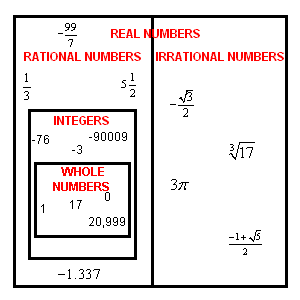# Real Numbers

The real numbers R are "all the numbers" on the number line . They include the rationals and irrationals together.

Even though real numbers are basic to all mathematics, to give a correct definition of the real numbers is a little bit advanced. If you've studied limits, the real numbers are the set of all possible limits of convergent sequences of rational numbers. If you haven't, don't worry about it yet; just think of them as the rationals plus the irrationals.

There are infinitely many real numbers, just as there are infinitely many rational or natural numbers , but it can be proved that the infinity of the real numbers is, in some way, a bigger infinity!

There are even "bigger" infinities, but you should take a set theory class for that!

The Venn diagram below shows the relationships of the various sets of numbers.